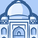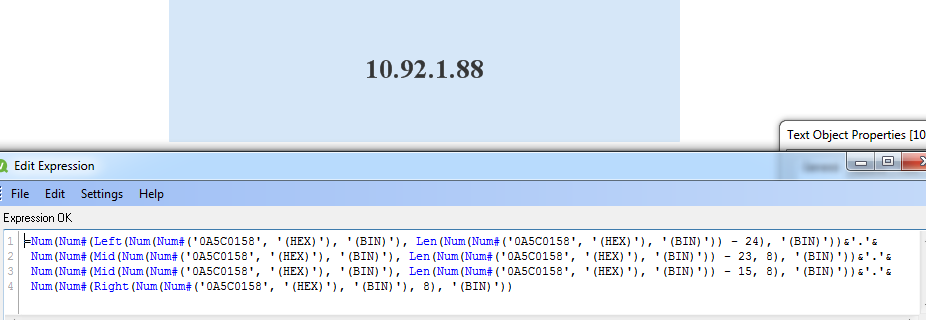# QlikView App Dev

Discussion Board for collaboration related to QlikView App Development.

Announcements
Join this live chat April 6, 10AM EST - QlikView to Qlik Sense REGISTER
cancel
Showing results for
Did you mean:Contributor III

## Convert HEX value to IP address

Hello,

On my chart, I have a column "SourceIP" with Hexadecimal value, like "0A5C0158", if I convert manualy this value to decimal I obtain 10.92.1.88 now I want to know if there are a possibility to convert all value in my chart from HEX to IP address, someone have an idea?

1 Solution

Accepted SolutionsMVP

You def. don't want to use static value in your chart, that was just an example. This is what you need:

=Num(Num#(Left(Num(Num#(SourceIP, '(HEX)'), '(BIN)'), Len(Num(Num#(SourceIP, '(HEX)'), '(BIN)')) - 24), '(BIN)'))&'.'&

Num(Num#(Mid(Num(Num#(SourceIP, '(HEX)'), '(BIN)'), Len(Num(Num#(SourceIP, '(HEX)'), '(BIN)')) - 23, 8), '(BIN)'))&'.'&

Num(Num#(Mid(Num(Num#(SourceIP, '(HEX)'), '(BIN)'), Len(Num(Num#(SourceIP, '(HEX)'), '(BIN)')) - 15, 8), '(BIN)'))&'.'&

Num(Num#(Right(Num(Num#(SourceIP, '(HEX)'), '(BIN)'), 8), '(BIN)'))

10 RepliesMVP

May be this:

=Num(Num#(Left(Num(Num#('0A5C0158', '(HEX)'), '(BIN)'), Len(Num(Num#('0A5C0158', '(HEX)'), '(BIN)')) - 24), '(BIN)'))&'.'&

Num(Num#(Mid(Num(Num#('0A5C0158', '(HEX)'), '(BIN)'), Len(Num(Num#('0A5C0158', '(HEX)'), '(BIN)')) - 23, 8), '(BIN)'))&'.'&

Num(Num#(Mid(Num(Num#('0A5C0158', '(HEX)'), '(BIN)'), Len(Num(Num#('0A5C0158', '(HEX)'), '(BIN)')) - 15, 8), '(BIN)'))&'.'&

Num(Num#(Right(Num(Num#('0A5C0158', '(HEX)'), '(BIN)'), 8), '(BIN)'))Contributor III
Author

Hello,

Thank you for your reply but it's works only for value  '0A5C0158' but not for other value in my chart.

I have a column named "SourceIP" all value are in HEX and I want to convert there défirent value to IP addess.MVP

Can you share few other values for where it isn't working?Contributor III
Author

For example the value 0A5C0A64 (10.92.10.100) have same IP (10.92.1.88) like the value '0A5C0158'MVP

Not sure what the issue is. It seem to work for both the values for me:MVP

Not sure where you are doing this, but replace all the red places with your new Hex Code:

=Num(Num#(Left(Num(Num#('0A5C0158', '(HEX)'), '(BIN)'), Len(Num(Num#('0A5C0158', '(HEX)'), '(BIN)')) - 24), '(BIN)'))&'.'&

Num(Num#(Mid(Num(Num#('0A5C0158', '(HEX)'), '(BIN)'), Len(Num(Num#('0A5C0158', '(HEX)'), '(BIN)')) - 23, 8), '(BIN)'))&'.'&

Num(Num#(Mid(Num(Num#('0A5C0158', '(HEX)'), '(BIN)'), Len(Num(Num#('0A5C0158', '(HEX)'), '(BIN)')) - 15, 8), '(BIN)'))&'.'&

Num(Num#(Right(Num(Num#('0A5C0158', '(HEX)'), '(BIN)'), 8), '(BIN)'))Contributor III
Author

Yes, If I replace '0A5C0158' by '0A5C0A64' it's works but all value in my chart have the same IP address '10.92.10.100'

Maybe the solution, it's to split the column "SourceIP" (AABBCCDD)

To have AA in new field like "HEXIP1"

BB in new field like "HEXIP2"

CC in new field like "HEXIP3"

DD in new field like "HEXIP4"

And after convert HEXIP1, HEXIP2, HEXIP3 and HEXIP4 from Hex to decimal to new other field as DECIP1, DECIP2, DECIP3, DECIP4

And to finish concatenate DECIP1+DECIP2+DECIP3+ECIP4 to have the final result

DECIP1.ECIP2.DECIP3.DCIP4MVP

You def. don't want to use static value in your chart, that was just an example. This is what you need:

=Num(Num#(Left(Num(Num#(SourceIP, '(HEX)'), '(BIN)'), Len(Num(Num#(SourceIP, '(HEX)'), '(BIN)')) - 24), '(BIN)'))&'.'&

Num(Num#(Mid(Num(Num#(SourceIP, '(HEX)'), '(BIN)'), Len(Num(Num#(SourceIP, '(HEX)'), '(BIN)')) - 23, 8), '(BIN)'))&'.'&

Num(Num#(Mid(Num(Num#(SourceIP, '(HEX)'), '(BIN)'), Len(Num(Num#(SourceIP, '(HEX)'), '(BIN)')) - 15, 8), '(BIN)'))&'.'&

Num(Num#(Right(Num(Num#(SourceIP, '(HEX)'), '(BIN)'), 8), '(BIN)'))Contributor III
Author

Great, thank you very much, it works